# 几种广义的函数展开法在构建偏微分方程精确解中的文献综述与应用（G′/G2）-展开法、（exp）-展开法构建(2 + 1)维Boiti-Leon-Pempinelli方程精确解A Literature Review and Application of Sev Eral Generalized Function Expansion Methods in Constructing Exact Solutions of Partial Differential Equations（G′/G2）-Expansion Method,（exp）-Expansion Method Construction (2 + 1) Exact Solution of the Dimensional Boiti-Leon-Pempinelli Equation

• 全文下载: PDF(2133KB)    PP.1659-1674   DOI: 10.12677/AAM.2019.810196
• 下载量: 235  浏览量: 335   国家自然科学基金支持

First, the system gives（G/G2）-expansion method, F-expansion method, （exp）-expansion method, improved Kudryashov method, direct truncation method, to construct the literature review of the origin and research status of the exact solutions of partial differential equations. Next, the steps of constructing the exact solutions of the partial differential equations by the above five generalized function expansion methods are given in comparison. Finally, through the above five generalized （G/G2）-expansion method, （exp）-expansion method in the function expansion method constructs the exact solution of the (2 + 1)-dimensional Boiti-Leon-Pempinelli equation. The control variable method is used to analyze the influence of three variables on the exact solution in the (2 + 1)-dimensional Boiti-Leon-Pempinelli equation.

1. 引言

$\left(\frac{{G}^{\prime }}{{G}^{2}}\right)$ -展开法，是Li Wen-An等借助Chun Quanbian在文献  提出的基础之上，在文献  提出的 $\left(\omega /g\right)$ -展开法而形成的，并用 $\frac{{G}^{\prime }}{G}$ -展开法构建出了Vakhncnko方程的精确解，其后在文献  -  中， $\left(\frac{{G}^{\prime }}{{G}^{2}}\right)$ -展开法被运用在求解各种方程的精确解中。

F-展开法，2003年由Y.B. Zhou等在文献  中首次提出并构建出了一个耦合Kdv方程的精确解，之后王明亮，斯仁道尔吉在文献    使用F-展开法KdV方程的精确解与广义Nizhnik-Novikov-Veselov方程组的精确解。

$\left(exp\right)$ -展开法，是由Ji-Huan He和Xu-Hong Wu在文献  首次提出，在文献    ，分别使用 $\left(exp\right)$ -展开法构建出了Konno-Oono方程，(2 + 1)-维Sawada-Kotera(SK)方程与(3 + 1)-维非线性evolution方程的精确解。

Kudryashov方法，由Kudryashov NA于1988年在文献  中提出，之后Anonymous在文献中将其运用到构建偏微分方程的精确解中，之后文献    分别使用改进的Kudryashov方法构建出了high-order nonlinear Schrödinger方程，nonlinear transmission line方程与Fractional nonlinear evolution方程的一系列精确解。

$\left\{\begin{array}{l}{u}_{ty}-{\left({u}^{2}-{u}_{x}\right)}_{xy}-2{v}_{xxx}=0,\hfill \\ {v}_{t}-{v}_{xx}-2u{v}_{x}=0.\hfill \end{array}$ (1)

2. 方法描述

$P\left(u,{u}_{x}.{u}_{x},{u}_{t},{u}_{xx},{u}_{xy},{u}_{xt},{u}_{yt},\cdots \right)=0,$ (2)

$u\left(x,y,t\right)=u\left(\xi \right),\xi =kx+my-\omega t,$ (3)

$U\left(u,{u}^{\prime },{u}^{″},\cdots \right)=0,$ (4)

2.1. $\left(\frac{{G}^{\prime }}{{G}^{2}}\right)$ -展开法构建偏微分方程精确解描述

$u\left(\xi \right)=\underset{i=0}{\overset{n}{\sum }}{a}_{i}{\left(\frac{{G}^{\prime }}{{G}^{2}}\right)}^{i},$ (5)

${\left(\frac{{G}^{\prime }}{{G}^{2}}\right)}^{\prime }=\varphi +\phi {\left(\frac{{G}^{\prime }}{{G}^{2}}\right)}^{2},$ (6)

$\left\{\begin{array}{l}\frac{{G}^{\prime }}{{G}^{2}}=\sqrt{\frac{\varphi }{\phi }}\frac{{c}_{1}\mathrm{cos}\left(\sqrt{\varphi \phi }\xi \right)+{c}_{2}\mathrm{sin}\left(\sqrt{\varphi \phi }\xi \right)}{{c}_{1}\mathrm{sin}\left(\sqrt{\varphi \phi }\xi \right)-{c}_{2}\mathrm{cos}\left(\sqrt{\varphi \phi }\xi \right)},\varphi \phi >0;\hfill \\ \frac{{G}^{\prime }}{{G}^{2}}=\sqrt{|\frac{\varphi }{\phi }|}\frac{{c}_{1}\mathrm{sinh}\left(\sqrt{|\varphi \phi |}\xi \right)+{c}_{2}\mathrm{cosh}\left(\sqrt{|\varphi \phi |}\xi \right)}{{c}_{1}\mathrm{cosh}\left(\sqrt{|\varphi \phi |}\xi \right)+{c}_{2}\mathrm{sinh}\left(\sqrt{|\varphi \phi |}\xi \right)},\varphi \phi <0;\hfill \\ \frac{{G}^{\prime }}{{G}^{2}}=-\frac{{c}_{1}}{\phi \left({c}_{1}\xi +{c}_{2}\right)},\varphi =0,\phi \ne 0,\hfill \end{array}$ (7)

2.2. F-展开法构建偏微分方程精确解描述

$u\left(\xi \right)=\underset{k=0}{\overset{n}{\sum }}{a}_{k}{F}^{K}\left(\xi \right),{a}_{n}\ne 0,$ (8)

$\left\{\begin{array}{l}{{F}^{\prime }}^{2}\left(\xi \right)={q}_{0}+{q}_{1}{F}^{2}\left(\xi \right)+{q}_{2}{F}^{4}\left(\xi \right),\\ {F}^{\prime }\left(\xi \right){F}^{″}\left(\xi \right)={q}_{1}F\left(\xi \right){F}^{\prime }\left(\xi \right)+2{q}_{2}{F}^{3}\left(\xi \right){F}^{\prime }\left(\xi \right),\\ {F}^{″}\left(\xi \right)={q}_{1}F\left(\xi \right)+2{q}_{2}{F}^{3}\left(\xi \right),\\ {F}^{‴}\left(\xi \right)={q}_{1}{F}^{\prime }\left(\xi \right)+6{q}_{2}{F}^{2}\left(\xi \right){F}^{\prime }\left(\xi \right).\end{array}$ (9)

2.3. (exp)-展开法构建偏微分方程精确解描述

$u\left(\xi \right)=\underset{n=0}{\overset{N}{\sum }}{a}_{n}{\left(\mathrm{exp}\left(-\varphi \left(\xi \right)\right)\right)}^{n}$ (10)

${\varphi }^{\prime }\left(\xi \right)=\mathrm{exp}\left(-\varphi \left(\xi \right)\right)+\mu \mathrm{exp}\left(\varphi \left(\xi \right)\right)+\lambda ,$ (11)

$\varphi \left(\xi \right)=\left\{\begin{array}{l}\mathrm{ln}\left(\frac{-\sqrt{{\lambda }^{2}-4\mu }\mathrm{tanh}\left(\frac{\sqrt{{\lambda }^{2}-4\mu }}{2}\left(\xi +c\right)-\lambda \right)}{2\mu }\right),{\lambda }^{2}-4\mu >0,\mu \ne 0,\hfill \\ \mathrm{ln}\left(\frac{-\sqrt{4\mu -{\lambda }^{2}}\mathrm{tanh}\left(\frac{\sqrt{{\lambda }^{2}-4\mu }}{2}\left(\xi +c\right)-\lambda \right)}{2\mu }\right),{\lambda }^{2}-4\mu <0,\mu \ne 0,\hfill \\ \mathrm{ln}\left(\frac{\lambda }{\mathrm{sinh}\left(\lambda \left(\xi +c\right)\right)+\mathrm{cosh}\left(\lambda \left(\xi +c\right)\right)-1}\right),{\lambda }^{2}-4\mu >0,\mu \ne 0,\lambda \ne 0,\hfill \\ \mathrm{ln}\left(-\frac{2\left(\lambda \left(\epsilon +c\right)\right)+2}{{\lambda }^{2}\left(\xi +c\right)}\right),{\lambda }^{2}-4\mu >0,\mu =0,\lambda \ne 0,\hfill \\ \mathrm{ln}\left(\xi +c\right),{\lambda }^{2}-4\mu =0,\mu =0,\lambda =0,\hfill \end{array}$ (12)

2.4. 改进的Kudryashov方法构建偏微分方程精确解描述

$u\left(\xi \right)=\underset{n=0}{\overset{N}{\sum }}{a}_{k}{\left(\varphi \left(\xi \right)\right)}^{n}$ (13)

$\varphi \left(\xi \right)=\frac{1}{1+c\mathrm{exp}\left(\xi \right)},$ (14)

$\varphi \left(\xi \right)$ 满足：

${\varphi }^{\prime }\left(\xi \right)-{\varphi }^{2}\left(\xi \right)+\varphi \left(\xi \right)=0,$ (15)

$\varphi \left(\xi \right)=\left\{\begin{array}{l}\frac{1}{2}\left(1-\mathrm{tanh}\left(\frac{\xi }{2}-\frac{\xi \mathrm{ln}{\xi }_{0}}{2}\right)\right),{\xi }_{0}>0,\hfill \\ \frac{1}{2}\left(1-\mathrm{coth}\left(\frac{\xi }{2}-\frac{\xi \mathrm{ln}{\xi }_{0}}{2}\right)\right),{\xi }_{0}>0,\hfill \end{array}$ (16)

$\left\{\begin{array}{l}{u}^{\prime }\left(\xi \right)=n\underset{n=0}{\overset{N}{\sum }}{a}_{n}{\left[\varphi \left(\xi \right)\right]}^{n}\left(\varphi \left(\xi \right)-1\right),\\ {u}^{″}\left(\xi \right)=n\underset{n=0}{\overset{N}{\sum }}{a}_{n}{\left[\varphi \left(\xi \right)\right]}^{n}\left(\varphi \left(\xi \right)-1\right)\left[\left(1+n\right)\varphi \left(\xi \right)-n\right].\end{array}$ (17)

2.5. 直接截断法构建偏微分方程精确解描述

$u\left(x,t\right)=\varphi \left(\xi \right){\text{e}}^{i\left({k}_{1}x-{\omega }_{1}t\right)},$ (18)

$\varphi \left(\xi \right)=\frac{\underset{i+j=0}{\overset{\tau }{\sum }}{a}_{ij}{f}^{i}\left(\xi \right){g}^{j\left(\xi \right)}}{{\left(qf\left(\xi \right)+rg\left(\xi \right)+p\right)}^{\tau }},$ (19)

$f\left(\xi \right),g\left(\xi \right)$ 满足以下椭圆函数条件：

$\left\{\begin{array}{l}f{\left(\xi \right)}^{2}+g{\left(\xi \right)}^{2}=1,\\ g\left(\xi \right)\sqrt{1-{k}^{2}f{\left(\xi \right)}^{2}}={f}^{\prime }\left(\xi \right),\\ -f\left(\xi \right)\sqrt{1-{k}^{2}f{\left(\xi \right)}^{2}}={g}^{\prime }\left(\xi \right).\end{array}$ (20)

3. 应用两种广义的函数展开法构建构建(2 + 1)维Boiti-Leon-Pempinelli方程精确解

$u\left(x,y,t\right)=u\left(\epsilon \right),\nu \left(x,y,t\right)=\nu \left(\xi \right),\epsilon =x+y-\omega t,$ (21)

${u}^{″}-2{u}^{3}-3\omega {u}^{2}-{\omega }^{2}u=0,$ (22)

3.1. $\left(\frac{{G}^{\prime }}{{G}^{2}}\right)$ -展开法构建(2 + 1)维Boiti-Leon-Pempinelli方程精确解

$m=1$ 则式(22)有如下形式的解

$u\left(\xi \right)={a}_{0}+{a}_{1}\left(\frac{{G}^{\prime }}{{G}^{2}}\right)$ (23)

$\left\{\begin{array}{l}{u}^{2}\left(\xi \right)={a}_{0}^{2}+{a}_{1}^{2}{\left(\frac{{G}^{\prime }}{{G}^{2}}\right)}^{2}+2{a}_{0}{a}_{1}\left(\frac{{G}^{\prime }}{{G}^{2}}\right),\hfill \\ {u}^{″}\left(\xi \right)=2{a}_{1}{\phi }^{2}{\left(\frac{{G}^{\prime }}{{G}^{2}}\right)}^{3}+2{a}_{1}\varphi \phi \left(\frac{{G}^{\prime }}{{G}^{2}}\right),\hfill \\ {u}^{3}\left(\xi \right)={a}_{1}^{3}{\left(\frac{{G}^{\prime }}{{G}^{2}}\right)}^{3}+3{a}_{1}^{2}{a}_{0}{\left(\frac{{G}^{\prime }}{{G}^{2}}\right)}^{2}+3{a}_{1}{a}_{0}^{2}\left(\frac{{G}^{\prime }}{{G}^{2}}\right)+{a}_{0}^{3}.\hfill \end{array}$ (24)

$u\left(\xi \right),{u}^{\prime }\left(\xi \right),{u}^{″}\left(\xi \right)$${u}^{3}\left(\xi \right)$ 代入方程(22)中，并平衡 ${\left(\frac{{G}^{\prime }}{{G}^{2}}\right)}^{i}\left(i=0,1,\cdots \right)$，并令各幂次的系数为零，得到关于 ${a}_{-1},{a}_{0},{a}_{1},\omega ,\varphi ,\phi$ 的代数方程组：

$\left\{\begin{array}{l}{\left(\frac{{G}^{\prime }}{{G}^{2}}\right)}^{3}:-2{a}_{1}{\phi }^{2}-2{a}_{1}^{3}=0,\hfill \\ {\left(\frac{{G}^{\prime }}{{G}^{2}}\right)}^{2}:-3\omega {a}_{1}^{2}-6{a}_{1}^{2}{a}_{0}=0,\hfill \\ {\left(\frac{{G}^{\prime }}{{G}^{2}}\right)}^{1}:2{a}_{1}^{2}\varphi \phi -6{a}_{1}{a}_{0}^{2}-6\omega {a}_{0}{a}_{1}-{\omega }^{2}{a}_{1}=0,\hfill \\ {\left(\frac{{G}^{\prime }}{{G}^{2}}\right)}^{0}:-{\omega }^{2}{a}_{0}-3\omega {a}_{0}^{2}-2{a}_{0}^{3}=0.\hfill \end{array}$ (25)

${a}_{0}=-\sqrt{\varphi \phi },{a}_{1}=±\phi ,\omega =2\sqrt{\varphi \phi }.$ (26)

$\varphi \phi >0$，得到三角函数解：

${u}_{\left(1,1\right)}\left(\xi \right)=-\sqrt{\varphi \phi }±\phi \left[\sqrt{\frac{\varphi }{\phi }}\frac{{c}_{1}\mathrm{cos}\left(\sqrt{\varphi \phi }\xi \right)+{c}_{2}\mathrm{sin}\left(\sqrt{\varphi \phi }\xi \right)}{{c}_{1}\mathrm{sin}\left(\sqrt{\varphi \phi }\xi \right)-{c}_{2}\mathrm{cos}\left(\sqrt{\varphi \phi }\xi \right)}\right].$ (27)

$\varphi \phi <0$，得到双曲函数解：

${u}_{\left(1,2\right)}\left(\xi \right)=-\sqrt{\varphi \phi }±\phi \left[\sqrt{|\frac{\varphi }{\phi }|}\frac{{c}_{1}\mathrm{sinh}\left(\sqrt{|\varphi \phi |}\xi \right)+{c}_{2}\mathrm{cosh}\left(\sqrt{|\varphi \phi |}\xi \right)}{{c}_{1}\mathrm{cosh}\left(\sqrt{|\varphi \phi |}\xi \right)+{c}_{2}\mathrm{sinh}\left(\sqrt{|\varphi \phi |}\xi \right)}\right].$ (28)

$\varphi =0,\phi \ne 0$，得到有理函数解：

${u}_{\left(1,3\right)}\left(\xi \right)=-\sqrt{\varphi \phi }\mp \phi \left[\frac{{c}_{1}}{\phi \left({c}_{1}\xi +{c}_{2}\right)}\right].$ (29)

${a}_{0}=\sqrt{\varphi \phi },{a}_{1}=±\phi ,\omega =-2\sqrt{\varphi \phi }.$ (30)

$\varphi \phi >0$，得到三角函数解：

${u}_{\left(1,4\right)}\left(\xi \right)=\sqrt{\varphi \phi }±\phi \left[\sqrt{\frac{\varphi }{\phi }}\frac{{c}_{1}\mathrm{cos}\left(\sqrt{\varphi \phi }\xi \right)+{c}_{2}\mathrm{sin}\left(\sqrt{\varphi \phi }\xi \right)}{{c}_{1}\mathrm{sin}\left(\sqrt{\varphi \phi }\xi \right)-{c}_{2}\mathrm{cos}\left(\sqrt{\varphi \phi }\xi \right)}\right].$ (31)

$\varphi \phi <0$，得到双曲函数解：

${u}_{\left(1,5\right)}\left(\xi \right)=\sqrt{\varphi \phi }±\phi \left[\sqrt{|\frac{\varphi }{\phi }|}\frac{{c}_{1}\mathrm{sinh}\left(\sqrt{|\varphi \phi |}\xi \right)+{c}_{2}\mathrm{cosh}\left(\sqrt{|\varphi \phi |}\xi \right)}{{c}_{1}\mathrm{cosh}\left(\sqrt{|\varphi \phi |}\xi \right)+{c}_{2}\mathrm{sinh}\left(\sqrt{|\varphi \phi |}\xi \right)}\right].$ (32)

$\varphi =0,\phi \ne 0$，得到有理函数解：

${u}_{\left(1,6\right)}\left(\xi \right)=\sqrt{\varphi \phi }\mp \phi \left[\frac{{c}_{1}}{\phi \left({c}_{1}\xi +{c}_{2}\right)}\right].$ (33)

3.2. (exp)-展开法构建(2 + 1)维Boiti-Leon-Pempinelli方程精确解

$u\left(\xi \right)={a}_{0}+{a}_{1}\mathrm{exp}\left(-\varphi \left(\xi \right)\right)$ (34)

$\left\{\begin{array}{l}{u}^{2}\left(\xi \right)={a}_{0}^{2}+{a}_{1}^{2}{e}^{-2\varphi \left(\xi \right)}+2{a}_{0}{a}_{1}{e}^{-\varphi \left(\xi \right)},\hfill \\ {u}^{″}\left(\xi \right)=\left[2{a}_{1}{\text{e}}^{-2\varphi \left(\xi \right)}+\lambda {a}_{1}{\text{e}}^{-\varphi \left(\xi \right)}\right]\left[{\text{e}}^{-\varphi \left(\xi \right)}+\mu {\text{e}}^{\varphi \left(\xi \right)}+\lambda \right],\hfill \\ {u}^{3}\left(\xi \right)={a}_{1}^{3}{\text{e}}^{-3\varphi \left(\xi \right)}+3{a}_{1}^{2}{a}_{0}{\text{e}}^{-2\varphi \left(\xi \right)}+3{a}_{1}{a}_{0}^{2}{\text{e}}^{-\varphi \left(\xi \right)}+{a}_{0}^{3}.\hfill \end{array}$ (35)

$u\left(\xi \right),{u}^{2}\left(\xi \right),{u}^{″}\left(\xi \right)$${u}^{3}\left(\xi \right)$ 代入方程(22)中，并合并 $\mathrm{exp}{\left(-\varphi \left(\xi \right)\right)}^{n},\left(n=0,1,2,3,\cdots \right)$ 相同项的系数，并令相同项系数为零，得到关于 ${a}_{0},{a}_{1},\cdots ,\mu ,\lambda ,\omega$ 的代数方程组：

$\left\{\begin{array}{l}\left({\text{e}}^{-3\varphi \left(\xi \right)}\right):2{a}_{1}-2{a}_{1}^{3}=0,\hfill \\ \left({\text{e}}^{-2\varphi \left(\xi \right)}\right):-3\omega {a}_{1}^{2}-6{a}_{1}^{2}{a}_{0}+3\lambda {a}_{1}=0,\hfill \\ \left({\text{e}}^{-1\varphi \left(\xi \right)}\right):2\mu {a}_{1}+{\lambda }^{2}{a}_{1}-6{a}_{1}{a}_{0}^{2}-6\omega {a}_{0}{a}_{1}-{\omega }^{2}{a}_{1}=0,\hfill \\ \left({\text{e}}^{0}\right):{\left(\frac{{G}^{\prime }}{{G}^{2}}\right)}^{0}:-{\omega }^{2}{a}_{0}-3\omega {a}_{0}^{2}-2{a}_{0}^{3}+\lambda \mu {a}_{1}=0.\hfill \end{array}$ (36)

${a}_{0}=-\sqrt{\mu },{a}_{1}=±1,\omega =2\sqrt{\mu }.$ (37)

${\lambda }^{2}-4\mu >0,\mu \ne 0$

${u}_{\left(2,1\right)}\left(\xi \right)=-\sqrt{\mu }±\mathrm{ln}\left(\frac{-\sqrt{{\lambda }^{2}-4\mu }\mathrm{tanh}\left(\frac{\sqrt{{\lambda }^{2}-4\mu }}{2}\left(\xi +c\right)-\lambda \right)}{2\mu }\right).$ (40)

${\lambda }^{2}-4\mu <0,\mu \ne 0$

${u}_{\left(2,2\right)}\left(\xi \right)=-\sqrt{\mu }±\mathrm{ln}\left(\frac{-\sqrt{4\mu -{\lambda }^{2}}\mathrm{tanh}\left(\frac{\sqrt{{\lambda }^{2}-4\mu }}{2}\left(\xi +c\right)-\lambda \right)}{2\mu }\right).$ (41)

$\mu \ne 0,\lambda \ne 0$

${u}_{\left(2,3\right)}\left(\xi \right)=-\sqrt{\mu }\mp \mathrm{ln}\left(\frac{\lambda }{\mathrm{sinh}\left(\lambda \left(\xi +c\right)\right)+\mathrm{cosh}\left(\lambda \left(\xi +c\right)\right)-1}\right).$ (42)

${\lambda }^{2}-4\mu >0,\mu =0,\lambda \ne 0$

${u}_{\left(2,4\right)}\left(\xi \right)=-\sqrt{\mu }±\mathrm{ln}\left(-\frac{2\left(\lambda \left(\epsilon +c\right)\right)+2}{{\lambda }^{2}\left(\xi +c\right)}\right).$ (43)

${\lambda }^{2}-4\mu =0,\mu =0,\lambda =0$

${u}_{\left(2,5\right)}\left(\xi \right)=-\sqrt{\mu }±\mathrm{ln}\left(\xi +c\right).$ (44)

${a}_{0}=\sqrt{\mu },{a}_{1}=±1,\omega =-2\sqrt{\mu }.$ (45)

${\lambda }^{2}-4\mu >0,\mu \ne 0$

${u}_{\left(2,6\right)}\left(\xi \right)=\sqrt{\mu }±\mathrm{ln}\left(\frac{-\sqrt{{\lambda }^{2}-4\mu }\mathrm{tanh}\left(\frac{\sqrt{{\lambda }^{2}-4\mu }}{2}\left(\xi +c\right)-\lambda \right)}{2\mu }\right).$ (46)

${\lambda }^{2}-4\mu <0,\mu \ne 0$

${u}_{\left(2,7\right)}\left(\xi \right)=\sqrt{\mu }±\mathrm{ln}\left(\frac{-\sqrt{4\mu -{\lambda }^{2}}\mathrm{tanh}\left(\frac{\sqrt{{\lambda }^{2}-4\mu }}{2}\left(\xi +c\right)-\lambda \right)}{2\mu }\right).$ (47)

$\mu \ne 0,\lambda \ne 0$

${u}_{\left(2,8\right)}\left(\xi \right)=\sqrt{\mu }\mp \mathrm{ln}\left(\frac{\lambda }{\mathrm{sinh}\left(\lambda \left(\xi +c\right)\right)+\mathrm{cosh}\left(\lambda \left(\xi +c\right)\right)-1}\right).$ (48)

${\lambda }^{2}-4\mu >0,\mu =0,\lambda \ne 0$

${u}_{\left(2,9\right)}\left(\xi \right)=\sqrt{\mu }±\mathrm{ln}\left(-\frac{2\left(\lambda \left(\epsilon +c\right)\right)+2}{{\lambda }^{2}\left(\xi +c\right)}\right).$ (49)

${\lambda }^{2}-4\mu =0,\mu =0,\lambda =0$

${u}_{\left(2,10\right)}\left(\xi \right)=\sqrt{\mu }±\mathrm{ln}\left(\xi +c\right).$ (50)

4. 数学实验与精确解的图形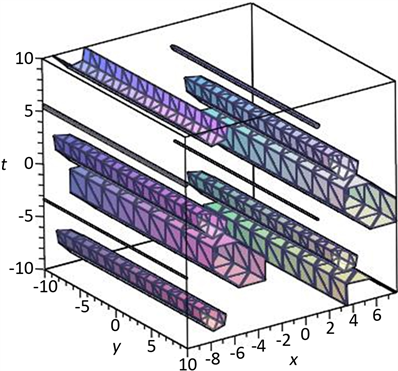Figure 1. ${u}_{\left(1,1\right)}\left(\xi \right)$ the x-y-t of 3D figures

${u}_{\left(1,1\right)}\left(\xi \right)$$\varphi =3,\phi =4,\omega =5,{c}_{1}=1,{c}_{2}=2$ 在x-o-t坐标系下(取 $y=1$ )的图形如图(2)，在x-o-y坐标系下(取 $t=1$ )的图形如图(3)。

${u}_{\left(1,1\right)}\left(\xi \right)$$\varphi =3,\phi =4,\omega =5,{c}_{1}=1,{c}_{2}=2$ 在y-o-t坐标系下(取 $x=1$ )的图形如图(4)，在x-y坐标系下(取 $t=1$ )的图形如图(5)。

${u}_{\left(1,1\right)}\left(\xi \right)$$\varphi =3,\phi =4,\omega =5,{c}_{1}=1,{c}_{2}=2$ 在x-t坐标系下(取 $y=1$ )的图形如图(6)，在y-t坐标系下(取 $x=1$ )的图形如图(7)。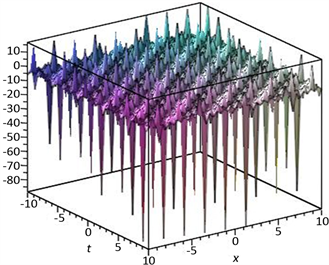Figure 2. ${u}_{\left(1,1\right)}\left(\xi \right)$ the x-o-t (t = 1) of 3D figures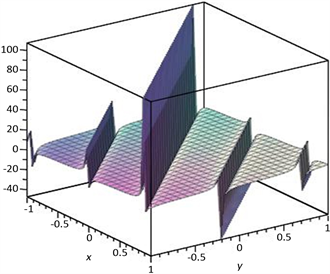Figure 3. ${u}_{\left(1,1\right)}\left(\xi \right)$ the x-o-y (t = 1) of 3D figures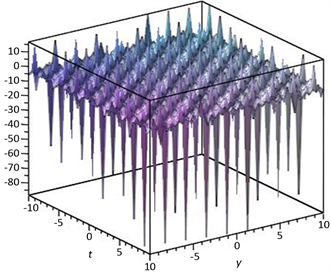Figure 4. ${u}_{\left(1,1\right)}\left(\xi \right)$ the y-o-t (t = 1) of 3D figures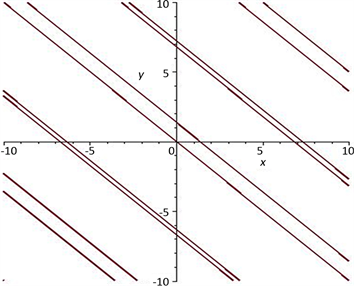Figure 5. ${u}_{\left(1,1\right)}\left(\xi \right)$ the x-y (t = 1) of figures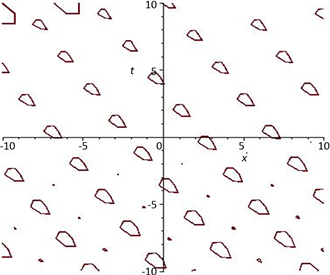Figure 6. ${u}_{\left(1,1\right)}\left(\xi \right)$ the x-t (t = 1) of figures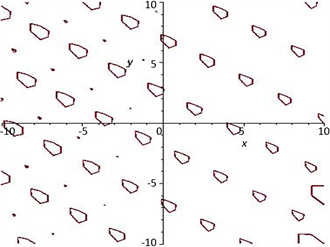Figure 7. ${u}_{\left(1,1\right)}\left(\xi \right)$ the x-t (t = 1) of figures

5. 结论

National Natural Science Foundation of China (国家自然科学基金，11371267)；Natural Science Foundation of Sichuan Province of China (四川省自然科学基金，2012ZA135)。

  Dodd, R.K. and Bullough, R.K. (1976) Bäcklund Transformations for the Sine-Gordon Equations. Proceedings of the Royal Society of London. Series A, Mathematical and Physical Sciences, 351, 499-523. https://doi.org/10.1098/rspa.1976.0154  Fan, E.G. and Zhang, H.Q. (1980) A New Approach to Bäcklund Transformations of Nonlinear Evolution Equations. Applied Mathematics and Mechanics, 19, 645-650. https://doi.org/10.1007/BF02452372  Fan, E.G. and Zhang, H.Q. (1980) Backlund Transformation and Exact Solutions for Whitham-Broer-Kaup Equations in Shallow Water. Applied Mathematics and Mechanics, 19, 713-716. https://doi.org/10.1007/BF02457745  El-Shiekh, R.M. (2018) Painlevé Test, Bäcklund Transformation and Consistent Riccati Expansion Solvability for Two Generalised Cylindrical Korteweg-de Vries Equations with Variable Coefficients. Zeitschrift für Naturforschung A, 73, 207-213.  Gomes, J.F., Retore, A.L., Spano, N.I. and Zimerman, A.H. (2015) Backlund Transformation for Integrable Hierarchies: Example-mKdV Hierarchy. Journal of Physics: Conference Series, 597, Article ID: 012039. https://doi.org/10.1088/1742-6596/597/1/012039  Matveev, V.B. (1979) Darboux Transformation and the Explicit Solutions of Differential-Difference and Difference-Difference Evolution Equations I. Letters in Mathematical Physics, 3, 217-222. https://doi.org/10.1007/BF00405296  Zhou, Z.X. (1988) On the Darboux Transformation for (1+2)-Dimensional Equations. Letters in Mathematical Physics, 16, 83-92. https://doi.org/10.1007/BF00398166  Huang, Y. (2013) Explicit Multi-Soliton Solutions for the KdV Equation by Darboux Transformation. IAENG International Journal of Applied Mathematics, 43, 135-137.  Qiu, D.Q. and Cheng, W.G. (2019) The N-Fold Darboux Transformation for the Kundu-Eckhaus Equation and Dynamics of the Smooth Position Solutions. Communications in Nonlinear Science and Numerical Simulation, 78, Article ID: 104887. https://doi.org/10.1016/j.cnsns.2019.104887  Shi, D.D. and Zhang, Y.F. (2020) Diversity of Exact Solutions to the Conformable Space-Time Fractional MEW Equation. Applied Mathematics Letters, 99, Article ID: 105994. https://doi.org/10.1016/j.aml.2019.07.025  范恩贵, 张鸿庆. 非线性孤子方程的齐次平衡法[J]. 物理学报, 1998, 47(3): 353-362.  范恩贵. 齐次平衡法、Weiss-Tabor-Carnevale法及Clarkson-Kruskal约化法之间的联系[J]. 物理学报, 2000, 49(8): 1409-1412.  Fan, E.G. (2000) Two New Applications of the Homogeneous Balance Method. Physics Letters A, 265, 353-357. https://doi.org/10.1016/S0375-9601(00)00010-4  Wei, Y., He, X.-D. and Yang, X.-F. (2016) The Homogeneous Balance of Undetermined Coefficients Method and Its Application. Open Mathematics, 14, 816-826. https://doi.org/10.1515/math-2016-0078  Stanbury, D.M. and Dean, H. (2019) Systematic Application of the Principle of Detailed Balancing to Complex Homogeneous Chemical Reaction Mechanisms. The Journal of Physical Chemistry A, 123, 5436-5445. https://doi.org/10.1021/acs.jpca.9b03771  Fan, E.G. and Zhang, J. (2002) Applications of the Jacobi Elliptic Function Method to Special-Type Nonlinear Equations. Physics Letters A, 305, 383-392. https://doi.org/10.1016/S0375-9601(02)01516-5  Feng, Z.S. (2002) On Explicit Exact Solutions to the Compound Burgers-KdV Equation. Physics Letters A, 293, 57-66. https://doi.org/10.1016/S0375-9601(01)00825-8  李林芳, 舒级, 文慧霞. 一类空时分数阶混合(1+1)维KdV方程的精确解[J]. 四川大学学报(自然科学版), 2018, 55(5): 912-916.  Arshed, S. and Arshad, L. (2019) Optical Soliton Solutions for Nonlinear Schrödinger Equation. Optik, 195, Article ID: 163077. https://doi.org/10.1016/j.ijleo.2019.163077  Li, W.A., Chen, H. and Zhang, G.C. (2009) The -Expansion Method and Its Application to Vakhnenko Equation. Chinese Physics B, 18, 400-404. https://doi.org/10.1088/1674-1056/18/2/004  Bian, C.Q., Pang, J., Jin, L.H. and Ying, X.M. (2010) Solving Two Fifth Order Strong Nonlinear Evolution Equations by Using the -Expansion Method. Communications in Nonlinear Science and Numerical Simulation, 15, 2337-2343.  冯庆江, 杨娟. 应用改进的-展开法求广义变系数Burgers方程的精确解[J]. 山西师范大学学报(自然科学版), 2016, 30(2): 7-11.  冯庆江, 雷学红. 应用-展开法求非线性发展方程的精确解(英文)[J]. 应用数学, 2013, 26(3): 538-543.  石兰芳, 聂子文. 应用全新-展开方法求解广义非线性Schrödinger方程和耦合非线性Schrödinger方程组[J]. 应用数学和力学, 2017, 38(5): 539-552.  冯庆江, 肖绍菊. 应用改进的-展开法求Zakharov方程的精确解[J]. 量子电子学报, 2015, 32(1): 40-45.  孙鹏, 崔泽建. 展开法及其在KPP方程中的应用[J]. 西华师范大学学报(自然科学版), 2015, 36(4): 336-338+381.  何姝琦, 陈立. 利用展开法求解KdV-Burgers方程和KdV-Burgers-Kuramoto方程[J]. 纺织高校基础科学学报, 2016, 29(3): 327-332.  王思源, 陈浩. 求解KdV方程和mKdV方程的新方法: 展开法[J]. 华南师范大学学报(自然科学版), 2014, 46(1): 42-45.  Zhou, Y.B., Wand, M.L. and Wand, Y.M. (2003) Periodic Wave Solutions to a Coupled KdV Equations with Variable Coefficients. Physics Letters A, 308, 31-36. https://doi.org/10.1016/S0375-9601(02)01775-9  张金良, 王明亮, 王跃明. 推广的F-展开法及变系数KdV和mKdV的精确解[J]. 数学物理学报, 2006(3): 353-360.  斯仁道尔吉. 通用F-展开法与nmKdV方程的精确解[J]. 内蒙古大学学报(自然科学版), 2018, 49(6): 561-566.  扎其劳, 斯仁道尔吉. 利用F-展开法解广义Nizhnik-Novikov-Veselov方程组(英文)[J]. 内蒙古大学学报(自然科学版), 2005(6): 14-19.  He, J.-H. and Wu, X.-H. (2006) Exp-Function Method for Nonlinear Wave Equations. Chaos, Solitons and Fractals, 30, 700-708. https://doi.org/10.1016/j.chaos.2006.03.020  Boz, A. and Bekir, A. (2008) Application of Exp-Function Method for (3 + 1)-Dimensional Nonlinear Evolution Equations. Computers and Mathematics with Applications, 56, 1451-1456.  Zayed, E.M.E., Amer, Y.A. and Al-Nowehy, A.-G. (2016) The Modified Simple Equation Method and the Multiple Exp-Function Method for Solving Nonlinear Fractional Sharma-Tasso-Olver Equation. Acta Mathematicae Applicatae Sinica, 32, 793-812. https://doi.org/10.1007/s10255-016-0590-9  Shakeel, M., Mohyud-Din, S.T. and Iqbal, M.A. (2018) Modified Extended Exp-Function Method for a System of Nonlinear Partial Differential Equations Defined by Seismic Sea Waves. Pramana, 91, 28. https://doi.org/10.1007/s12043-018-1601-6  Öziş, T. and Köroğlu, C. (2008) A Novel Approach for Solving the Fisher Equation Using Exp-Function Method. Physics Letters A, 372, 3836-3840. https://doi.org/10.1016/j.physleta.2008.02.074  Kudryashov, N.A. (1988) Exact Soliton Solutions of the Generalized Evolution Equation of Wave Dynamics. Journal of Applied Mathematics and Mechanics, 52, 360-365. https://doi.org/10.1016/0021-8928(88)90090-1  Taghizadeh, N., Mirzazadeh, M. and Mahmoodirad, A. (2013) Application of Kudryashov Method for High-Order Nonlinear Schrdinger Equation. Indian Journal of Physics, 87, 781-785. https://doi.org/10.1007/s12648-013-0296-2  Hubert, M.B., Betchewe, G., Doka, S.Y. and Crepin, K.T. (2014) Soliton Wave Solutions for the Nonlinear Transmission Line Using the Kudryashov Method and the -Expansion Method. Applied Mathematics and Computation, 239, 299-309. https://doi.org/10.1016/j.amc.2014.04.065  Ferdous, F. and Hafez, M.G. (2018) Oblique Closed form Solutions of Some Important Fractional Evolution Equations via the Modified Kudryashov Method Arising in Physical Problems. Journal of Ocean Engineering and Science, 3, 244-252. https://doi.org/10.1016/j.joes.2018.08.005  Zhao, D., Luo, H.-G., Wang, S.-J. and Zuo, W. (2004) A Direct Truncation Method for Finding Abundant Exact Solutions and Application to the One-Dimensional Higher-Order Schrodinger Equation. Chaos, Solitons and Fractals, 24, 533-547. https://doi.org/10.1016/j.chaos.2004.09.016  Zhao, D., Luo, H.G. and Chai, H.Y. (2008) Integrability of the Gross-Pitaevskii Equation with Feshbach Resonance Management. Physics Letters A, 372, 5644-5650. https://doi.org/10.1016/j.physleta.2008.07.013  Choudhary, A.K. and Nagar, S.K. (2013) Direct Truncation Method for Order Reduction of Discrete Interval System. 2013 Annual IEEE India Conference (INDICON), Mumbai, 13-15 December 2013.  刘转玲. Burgers方程的两种不同形式的精确解[J]. 陇东学院学报, 2014, 25(1): 13-15.  董长紫. Klein-Gordon方程的精确解[J]. 商丘师范学院学报, 2011, 27(3): 38-41.  董长紫. 高次非线性薛定谔微分方程的新精确解[J]. 唐山师范学院学报, 2010, 32(2): 32-34.  Khater, M.M.A., Seadawy, A.R. and Lu, D.C. (2017) Elliptic and Solitary Wave Solutions for Bogoyavlenskii Equations System, Couple Boiti-Leon-Pempinelli Equations System and Time-Fractional Cahn-Allen Equation. Results in Physics, 7, 2028-2035. https://doi.org/10.1016/j.rinp.2017.06.049  蔡珊珊, 周钰谦, 刘倩. (2+1)维Boiti-Leon-Pempinelli方程的椭圆函数周期波解[J]. 四川师范大学学报(自然科学版), 2015, 38(4): 504-507.  Fang, J.-P., Ren, Q.-B. and Zheng, C.-L. (2015) New Exact Solutions and Fractal Localized Structures for the (2+1)-Dimensional Boiti-Leon-Pempinelli System. Zeitschrift für Naturforschung A, 60, 245-251.  Zayed, E.M.E. (2009) The -Expansion Method and Its Applications to Some Nonlinear Evolution Equations in the Mathematical Physics. Journal of Applied Mathematics and Computing, 30, 89-103. https://doi.org/10.1007/s12190-008-0159-8  Boiti, M. and Leon, J. (1987) Spectral Transform for a Two Spatial Dimension Extension of the Dispersive Long Wave Equation. Inverse Problems, 3, 371-387. https://doi.org/10.1088/0266-5611/3/3/007  Huang, D.J. and Zhang, H.Q. (2004) Exact Traveling Wave Solutions for the Boiti-Leon-Pempinelli Equation. Chaos Solitons Fractals, 22, 243-247. https://doi.org/10.1016/j.chaos.2004.01.004  Lü, Z. and Zhang, H. (2004) Soliton-Like and Multi-Soliton Like Solutions of the Boiti-Leon-Pempinelli Equation. Chaos Solitons Fractals, 19, 527-531. https://doi.org/10.1016/S0960-0779(03)00104-8  Yomba, E. (2008) Construction of New Soliton-Like Solutions of the (2+1)-Dimensional Dispersive Long Wave Equation. Chaos Solitons Fractals, 20, 1135-1139. https://doi.org/10.1016/j.chaos.2003.09.026  Baskonus, H.M. and Bulut, H. (2016) Exponential Prototype Structures for (2 + 1)-Dimensional Boiti-Leon-Pempinelli Systems in Mathematical Physics. Waves in Random and Complex Media, 26, 189-196. https://doi.org/10.1080/17455030.2015.1132860  费金喜, 应颖洁, 雷燕. (2+1)维Boiti-Leon-Pempinelli方程系统的对称约化和精确解[J]. 丽水学院学报, 2014, 36(5): 8-14.